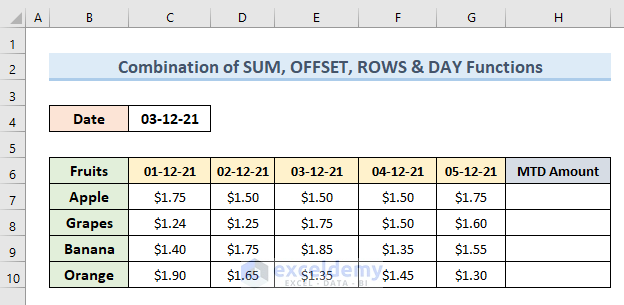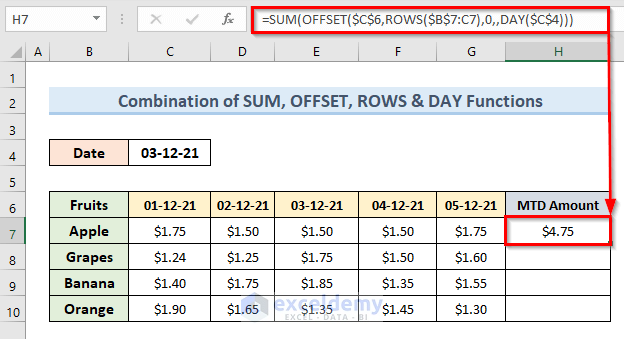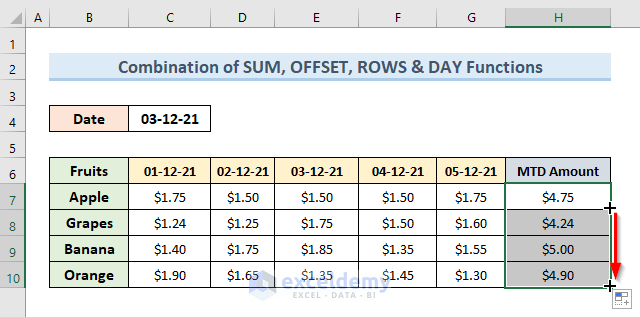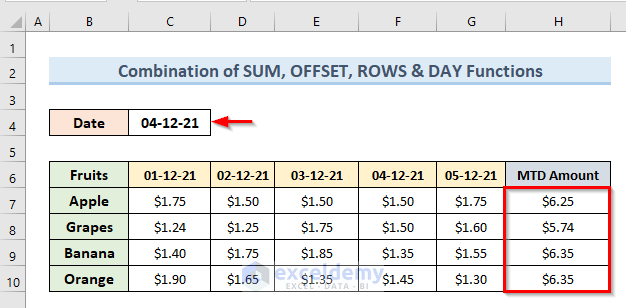# How to Calculate MTD (Month to Date) in Excel (3 Methods)

In this tutorial, we will demonstrate how to calculate MTD (Month to Date) in Excel. Microsoft Excel offers a variety of options for calculating MTD depending on several factors. After this article, you will be able to calculate MTD easily with different methods.

## What is MTD?

The term MTD refers to ‘Month to Date.’ It’s the time span from the start of the present month to the current time but not today’s date, as it may not yet be finished. MTD is used to provide information on a specific activity for a specific time period.

## 3 Easy Methods to Calculate MTD (Month to Date) in Excel

### 1. Combine SUM, OFFSET, ROWS & DAY Functions to Calculate MTD in Excel

Suppose we have the following dataset of a fruit stall. The dataset contains the sales amount of different fruits for the first five days of the month. Now, we want to know the Month to Date amount for each fruit. In the following example, we will calculate MTD up to the given date in cell C4. We will use a combination of SUM, OFFSET, ROWS, and DAY functions.

###Let’s see the steps to perform this action.

STEPS:

• To begin with, select cell H7. Type the following formula in that cell:
`=SUM(OFFSET(\$C\$6,ROWS(\$B\$7:C7),0,,DAY(\$C\$4)))`
• Then, press Enter.
• So, in cell H7, it will tell us the total sales value up to the date ‘3-12-21.’

###• After that drag the Fill Handle tool from cell to H10 to get results for other fruits.

###• Finally, change the date to ‘4-12-21’ from ‘3-12-21’. We can see that the MTD amount changes automatically.

###🔎 How Does the Formula Work?

• OFFSET(\$C\$6,ROWS(\$B\$7:C7),0,,DAY(\$C\$4)): This part returns the range. The range is specified for row 7 taking cell C6 and the date of cell C4 as references.
• SUM(OFFSET(\$C\$6,ROWS(\$B\$7:C7),0,,DAY(\$C\$4))): This part returns the sum of sales amount up to the date in cell C4.

### 2. Calculate MTD in Excel with SUMIF Function & Helper Column

In this method, we will calculate MTD with the SUMIF function. To do this we will have to add helper columns to our dataset. In the following dataset, we have sales data of a fruit stall. It gives us the sales amount for the first 10 days for different fruits. Let’s see the steps to calculate MTD from this dataset.

STEPS:

• First, insert two helper columns with the dataset.• Next, insert the following formula in cell E7:
`=IF(B7<\$C\$4,MONTH(\$C\$4),0)`
• Press, Enter.
• The above formula returns 12 in cell E7.Here, the IF function returns the value of the month of cell C4 in cell E7 if the value of B7 > C4. Otherwise, the formula will return 0.

• So, if we drag the formula to the end of the dataset we will get a result like the following image.• Again, insert the following formula in cell F7:
`=IF(MONTH(B7)=MONTH(\$C\$4),E7,0)`
• Press Enter.
• Then, drag the Fill Handle to the end of the dataset.
• So, we can see the results of the above commands in the following image.Here, in the above formula, the MONTH function gets the value of the month from the date in cells B7 and C4. With the IF formula, it returns the value of cell E7 if the value of B7 and C4 is equal. Otherwise, it will return 0.

• Furthermore, select cell H10. Input the following formula in that cell:
`=SUMIF(\$E\$7:\$E\$16,MONTH(\$C\$4),D7:D16)`
• Hit Enter.
• Finally, get the result in cell H10.Here, the SUMIF function returns the sum of the range (D7:D16). It is valid until the value by the MONTH function in cell C4 remains within the range (E7:E16).

NOTE:

Here, if we change the date value in cell C4 the MTD amount will change for the updated date accordingly.

### 3. Use Pivot Table & Slicer to Calculate MTD in Excel

Now, we will calculate MDT using a pivot table and a slicer. For this, we will use the given dataset of a fruit stall. The dataset includes fruit sales for the 31 days of December 2021 and the 15 days of January 2022.

In the following image, we have given a part of the dataset. To access the full dataset we will suggest you download the practice workbook added to this article.Let’s see the steps to do this method.

STEPS:

• Firstly, select any cell from the data range.
• Secondly, go to Insert > Table.
• Check the option ‘My table has headers’ and click on OK.• The dataset is now in table format.
• Thirdly, add a new column named Day in the dataset.
• Insert the following formula in cell E5:
`=DAY(B5)`
• Press Enter.
• Double-click on the Fill Handle icon or drag it to the end of the dataset.• The above commands give us the results like the below image.Here the DAY function returns the value of a day from a date field.

• Furthermore, select any cell from the data range. We have selected cell B4.
• Then, go to the Insert tab and select the option ‘PivotTable’.• A new dialogue box will open. Click on OK.• A section named ‘PivotTable Fields’ like the following image will open in a new worksheet.• Here, drag the fields Quarters & Years in the Rows section, Price field in the Value section, and Date field in the Columns section.
• After dragging those fields we will get results like the below image.• In the above image, we can not compare the sales amount of months December and January. Because the result of December month is for 30 days whereas for January it is 15 To compare these two only for 15 days we will add a slicer.
• Go to the ‘PivotTable Analyze’ tab and select the option ‘Insert Slicer’.• We will get results like the image below. Check the options Day from that dialogue box and click on OK.• As a result, we get a slicer for calculating days.• Now, from the excel ribbon input the value 7 in the Columns field.• So, we will get the slicer for all 30 It will look like a calendar.• In the end, select the first 15 days from the slicer. We can see that the table displays the sales data only for 15 days for both January and December months.## Conclusion

In conclusion, by following this tutorial we can easily calculate MTD in excel. To get the best result download the practice workbook added to this article and practice yourself. Feel free to comment in the below box if you face any problems or have any suggestions.

## Related Articles#### Mukesh Dipto

Hi there! I am Mukesh Dipto. I graduated with a bachelor's degree in engineering. Currently, I am working as a technical content writer in ExcelDemy. You will find all my articles on Microsoft Excel on this site. Outside of the workplace, my hobbies and interests include sports and meeting new people. I also enjoy sports. My favorite sports are football (to watch and play) and Badminton (play).

We will be happy to hear your thoughts5 Excel Hacks You Never Knew